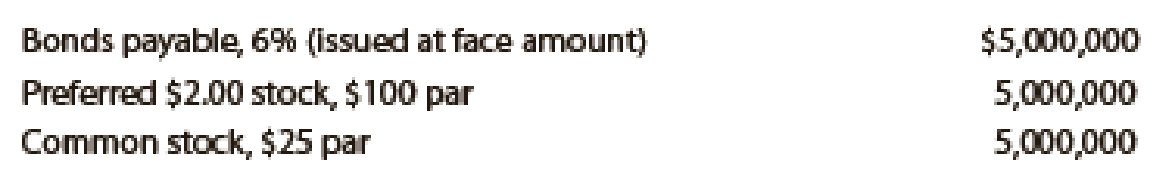# Domanico Co., which produces and sells biking equipment, is financed as follows: Income tax is estimated at 40% of income. Determine the earnings per share of common stock, assuming that the income before bond interest and income tax is (a) $600,000, (b)$800,000, and (c) $1,200,000.BuyFindarrow_forward ### Financial Accounting 15th Edition Carl Warren + 2 others Publisher: Cengage Learning ISBN: 9781337272124 #### Solutions Chapter SectionBuyFindarrow_forward ### Financial Accounting 15th Edition Carl Warren + 2 others Publisher: Cengage Learning ISBN: 9781337272124 Chapter 14, Problem 1E Textbook Problem 6 views ## Domanico Co., which produces and sells biking equipment, is financed as follows:Income tax is estimated at 40% of income.Determine the earnings per share of common stock, assuming that the income before bond interest and income tax is (a)$600,000, (b) $800,000, and (c)$1,200,000.

a)

To determine

Calculate earnings per share of common stock.

### Explanation of Solution

Bonds: Bonds are long-term promissory notes that are issued by a company while borrowing money from investors to raise fund for financing the operations.

Common stock: It refers to a security issued in a form of certificate and implies the right of ownership of an investor over a portion of company’s earnings and assets.

Earnings per Share: It is a portion of profit that is earned by each common stock.

Formula:

Earnings per share=Net income Preferred dividends Number of common shares outstanding

Determine Earnings per share of common stock, if income before bond interest and income tax is $600,000.  Particulars Net income before interest on bonds and income tax$600,000 Less: Interest on bonds $300,000(1) Income before income tax$300,000 Less: Income tax expense $120,000(2) Net income$180,000 Dividends on preferred stock $100,000(3) Available of dividends on common stock$80,000 Number of common stock outstanding ÷200,000(4) Earnings per share of common stock $0.40 Table (1) Working notes: (1) Calculate interest on bonds. Interest expense=Facevalueofbonds×Rate of Interest=$5,000,000×6%=\$300,000

(2)

Calculate income tax expense

b)

To determine

Calculate earnings per share of common stock.

c)

To determine

Calculate Earnings per share of common stock.

### Still sussing out bartleby?

Check out a sample textbook solution.

See a sample solution

#### The Solution to Your Study Problems

Bartleby provides explanations to thousands of textbook problems written by our experts, many with advanced degrees!

Get Started

Find more solutions based on key concepts
List and describe four determinants of a countrys productivity.

Brief Principles of Macroeconomics (MindTap Course List)

Why do public utilities generally use different capital structures than biotechnology companies?

Fundamentals of Financial Management, Concise Edition (MindTap Course List)

Operating cash flows rather than accounting income are listed in Table 12.1. Why do we focus on cash flows as o...

Fundamentals of Financial Management, Concise Edition (with Thomson ONE - Business School Edition, 1 term (6 months) Printed Access Card) (MindTap Course List)

PREPARE CHECK AND STUB Based on the following information, prepare a check and stub:

College Accounting, Chapters 1-27 (New in Accounting from Heintz and Parry)